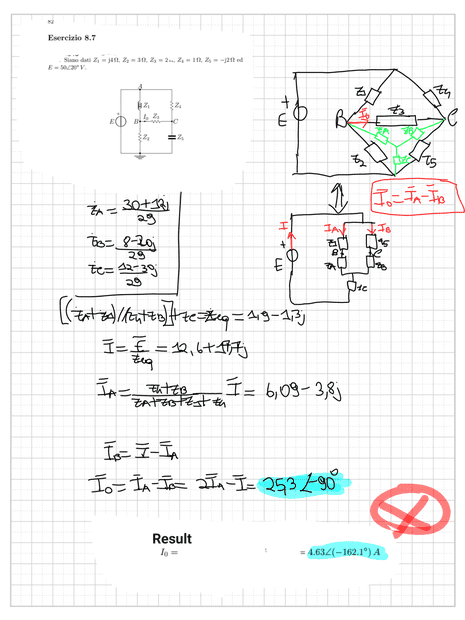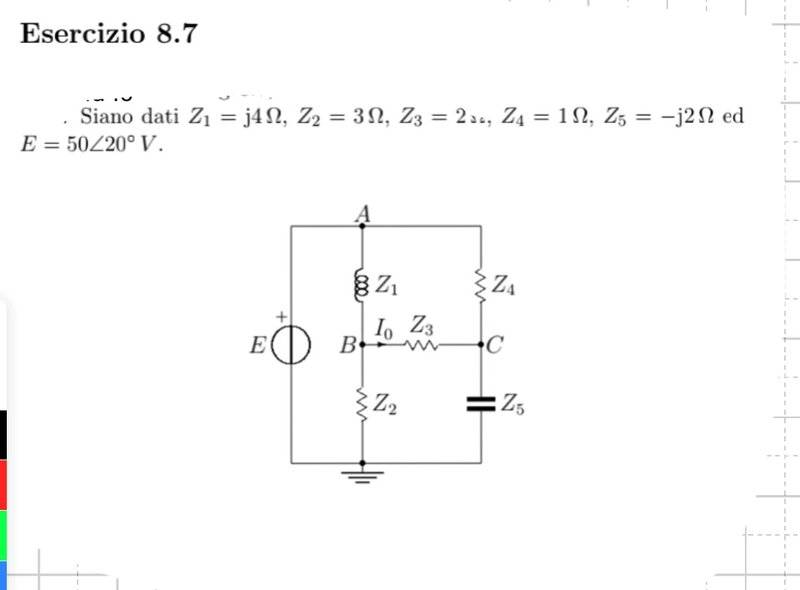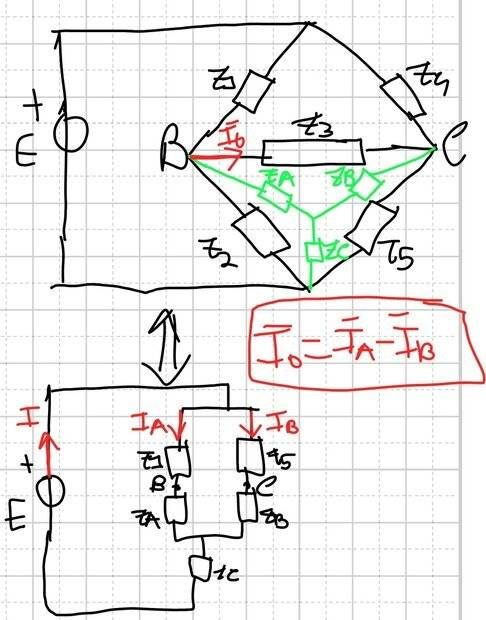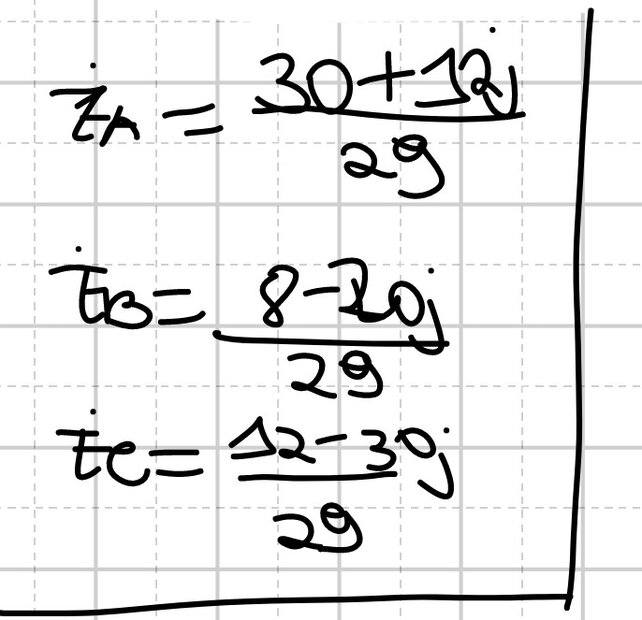# Current calculation of a Y-junction

• simphysics
In Summary, The person is trying to solve a circuit but there is something wrong. They made a delta-Y conversion and their new impedances are Z_A, Z_B, and Z_C. The current I0 that flows between nodes A and B is not accounted for in their equation. They need to find the potentials at points B and C and then find I_0.f

#### simphysics

Homework Statement
Find I0 in the following circuit
Relevant Equations
I used the delta-Y conversion
Hello everyone!
I've been trying to solve this circuit but there's something wrong. Firstly, I made a Delta-Y conversion so that1 and zA were in series, and so were z5 and zb. I really don't know if writing "I0=IA-IB" is correct, cause basically I0 is the current that flows between the nodes A and B. Can someone help me, please?
Thank you!It is possible that you are getting few answers because the drawing is nearly illegible. Not all of the circuit elements seem to be resistors either. So that scares me away. I can make out (I think) ##Z_1## and ##Z_2## on the left hand side, ##Z_3## in the middle and ##Z_4## and ##Z_5## on the right.

Then in the equivalent Y layout we have some other symbols that I cannot make out.

Type in your equations. Explain what they mean and what justifies them and we can talk.

Thank you.
I'll try to reupload the pics.
This is the circuit:I did a delta-Y conversionand the new impedances are:I hope that the rest is pretty legible.
Thank you, again

Much more legible, yes. Although the forum guidelines ask that you type in your work rather than import images.
Core post content should be typed out and not solely contained in an image, PDF, or other attachment. (Exceptions at Mentor's discretion.) Use images for supporting figures. Use LaTeX to type mathematical expressions and equations. (See: Learn LaTeX for Math Equations)
[emphasis mine]
With the images made legible, I can see that ##Z_1## is an inductor and ##Z_5## is a capacitor. So this is beyond what I am competent to analyze.

I can see that you've transformed to something that might be a taus (##\dot{\tau_A}##, ##\dot{\tau_B}## and ##\dot{\tau_C}##) with bars through the symbols. But those equations would be a lot prettier if rendered in ##\LaTeX##.

See the LaTeX guide that is linked here, in the quote above and which is also linked below the edit window when you respond.

I really don't know if writing "I0=IA-IB" is correct, cause basically I0 is the current that flows between the nodes A and B.
That doesn't seem correct (though I'm no expert). There is a junction between ##Z_A## and ##Z_B## and your equation doesn't take account of the current flowing from the junction into ##Z_C##.

I think you need to find the potentials at points B and C. This gives the potential difference between B and C (##V_{BC} = V_C - V_B##). Then ##I_0 = \frac {V_{BC}}{R_3}##.

Notes

A, B and C are the names of points in the circuit So calling the Y-impedances ##Z_A, Z_B## and ##Z_C## isn't a good idea!. Maybe something like ##Z_P, Z_Q## and ##Z_R## would be better.

Your handwriting is difficult to read. Have some sympathy for whoever is marking your work! (And if they can't read it, you may lose marks.) For posting here, you should use Latex for equations (not that hard) as already noted by @jbriggs444.